# Something wrong with kernel density estimation of uqlab

Hi everyone,

I am using kernel marginals to fit my given input data. Hoever, it appears that the uqlab cannot accurately model my data while the matlab function ksdensity can model it well. The following picture is the histogram of the input data.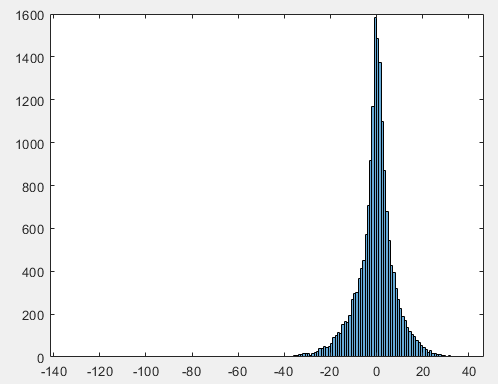The following code is what I have done in the uqlab:

Input.Marginals.Type = ‘KS’;
Input.Marginals.Parameters = Sample;
object = uq_createInput(Input);
X = uq_getSample(17520);

However, the histogram of X is quite different from the input data. Its histogram is as follows: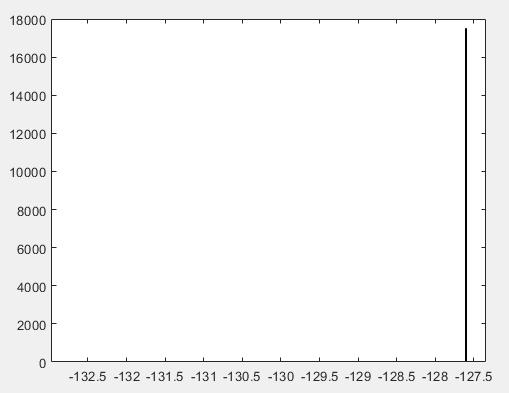And I also adopted the matlab function ksdensity to estimate the distribution, using the following code:

[f,xi] = ksdensity(Sample);
plot(xi,f)

The resulting density is simialr to the input data, which is as follows: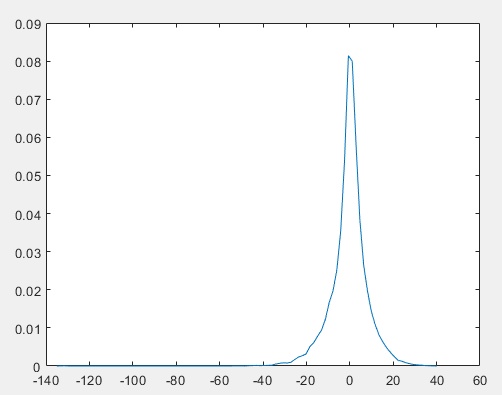So I am wondering why the uqlab kernal marginals give such different results?

Any help will be appreciated. Thank you very much.

Best regards,
Yx Wu

Your code is a little bit confusing to me. I am not sure if you are trying to construct your input or infer from the data. It might be helpful to start with the example: https://www.uqlab.com/input-inference-of-marginals

Thanks for your kind reply. Actually I an trying to use kernel estimation from my input data to construct an input marginal distribtuion.

Although the code runs smoothly, the estimated results do not fit my input data at all.
To be specific, I simulate some samples after creating an input object, however, these results deviate from my input data distribution as well as the results obtained from matlab function ksdensity. As you can see from the pictures above: the first one is the true data distribution, the second is the result obtained from uq_getSample, the third one is the result from ksdensity of matlab.

I thought that uqlab uses the matlab function ksdensity to conduct kernel estimation. Then why these results be so different? Thanks again.

What is the dimension of your data “Sample”?

It is one dimension with 17520 observations.

I tested the inference function of UQLab, and it seems to be working well. Since I cannot see any data, I can only suggest checking step-by-step, looking into the “Sample” and “Object” parameters, or starting from the example that UQLab provides, as they define the Input object differently. However, I believe that both ways will reach the same result.

Dear @YuanXi_Wu

As @142127 pointed out, it is very difficult to pinpoint the problem without having a minimal reproducible example. Can you share one?

Best regards
Styfen

Dear @styfen.schaer

Sorry for the late reply. I have attached the matlab data of the Sample in the Sample.zip. My codes for using uqlab and matlab bulit-in fitdist to do kernel density estimate and draw samples are as follows:

1. Uqlab
uqlab
Input.Marginals = uq_KernelMarginals(Sample);
object = uq_createInput(Input);
X=uq_getSample(object,10000);
histogram(X, ‘Normalization’,‘probability’)

2. MATLAB built-in fitdist function
pd = fitdist(Sample,‘kernel’);
Y = random(pd, [10000,1]);
histogram(Y, ‘Normalization’,‘probability’)

The resulting histograms of X and Y are as follows:

1. Histogram of X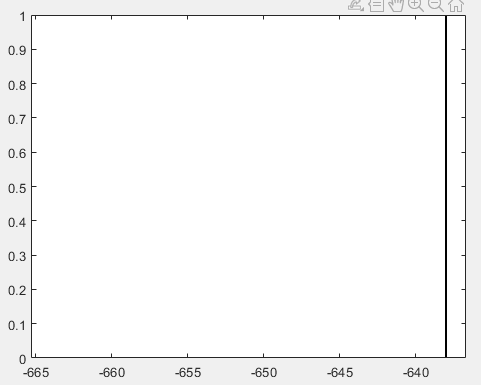1. Histogram of Y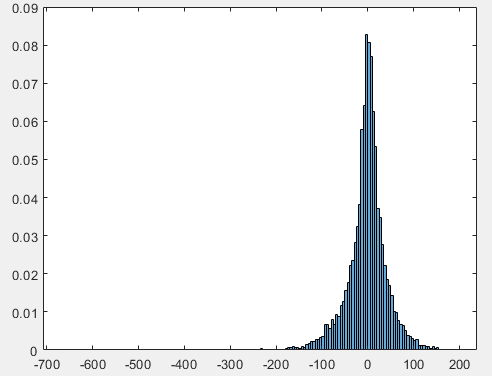Obviously, Y fits the original data Sample, while X is quite different. I s there anything wrong with my code?
Sample.zip (132.3 KB)

Dear @142127

Sorry for the late reply. I have attached my data and results in the above post. Thank you for your kind help.

Dear @YuanXi_Wu

Thank you for providing the data and pointing out this problem. We have investigated the issue and there is indeed a bug in UQLab. Fortunately, the problem is small and can be easily fixed.

Navigate to the following file in your UQLab installation:
`path_to_uqlab\modules\uq_input\builtin\uq_default_input\uq_initialize_uq_default_input.m`.
and then change line 100 from
`uBounds(2) = min(KSPar) + ks.Nstd*stdKSPar;`
to
`uBounds(2) = max(KSPar) + ks.Nstd*stdKSPar;`

Best regards
Styfen

3 Likes

Dear @styfen.schaer

Thank you for your attention and help. I can proceed my research now with your kind help.

Best regards,
Yuanxi Wu